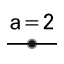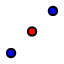# Visualizing the Angle Sum in a Triangle

Create an interactive figure that allows your students to explore the angle sum in a triangle.

## Instructions

 1Create a triangle ABC with counter clockwise orientation. 2Create the angles α, β and γ of triangle ABC. 3Create a slider for angle δ with Interval 0 ̊ to 180 ̊ and Increment 10 ̊. 4Create a slider for angle ε with Interval 0 ̊ to 180 ̊ and Increment 10 ̊. 5Create midpoint D of segment AC and midpoint E of segment AB. 6Rotate the triangle around point D by angle δ (setting clockwise). 7Rotate the triangle around point E by angle ε (setting counter clockwise). 8Move both sliders δ and ε to show 180 ̊.

## Instructions (continued)

 9Create angle ζ using the points A’C’B’. 10Create angle η using the points C'1B'1A'1. 11Enhance your construction using the Style Bar. Hint: Congruent angles should have the same color. 12Create dynamic text displaying the interior angles and their values (e.g. enter `α = `and select α from the list of objects on tabof the Advanced section). 13Calculate the angle sum by entering `sum = α + β + γ` in the Algebra Input. 14Insert the angle sum as a dynamic text: `α + β + γ =` and select sum from the list of objects on tab. 15Matchcolors of corresponding angles and text using the Style Bar. 16Fix all texts that are not supposed to be moved by using the Style Bar.